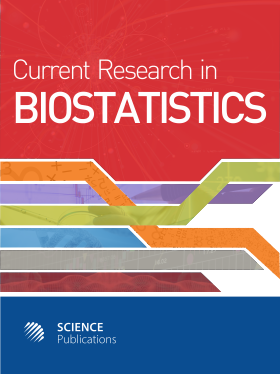Frequency: Continuous
ISSN: 2524-2210 (Print)
ISSN: 2524-2229 (Online)
Research Article Open Access

# Comparison of Probit and Logistic Regression Models in the Analysis of Dichotomous Outcomes

Amrutha Jose1, Mariyamma Philip1, Lavanya Tumkur Prasanna2 and M. Manjula1
• 1 National Institute of Mental Health and Neurosciences (NIMHANS), India
• 2 Lund University, Sweden

## Abstract

Probit and logistic regression models are members of the family of generalized linear models, used for estimating the functional relationship between the dichotomous dependent and independent variables. The current study is designed to find the performance of logistic and probit regression models in different conditions under multivariate normality. The objective of the study is to compare the performance of probit and logistic regression models under multivariate normal. A Monte Carlo simulation study was done in which artificial datasets were generated under multivariate normality. Datasets were generated by employing the latent variable approach, under different variance-covariance matrices, varying sample sizes and prevalences. For each of the combinations, 1000 simulations were carried out. Probit and logistic regression analyses were performed and compared using parameter estimates, standard error, Likelihood Ratio test, RMSEs, null and residual deviances, different pseudo R2 measures, AIC, BIC and Correct Percent Prediction. A live data set was also used to compare the efficiency of the models. It was evident from AIC, BIC and RMSE values that logit and probit models fit the dataset equally well in all the combinations of sample size, correlation structure and proportion of outcome. However, sensitivity, specificity and CPP values showed that the logit model predicts the outcome better than the probit model in most of the situations. The results showed that the probit and logit models perform equally well under multivariate normality.

Current Research in Biostatistics
Volume 10 No. 1, 2020, 1-19

DOI:

Submitted On: 29 January 2020 Published On: 26 March 2020

How to Cite: Jose, A., Philip, M., Prasanna, L. T. & Manjula, M. (2020). Comparison of Probit and Logistic Regression Models in the Analysis of Dichotomous Outcomes. Current Research in Biostatistics, 10(1), 1-19. https://doi.org/10.3844/amjbsp.2020.1.19

• 3,063 Views